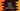# Python program to create a list of random numbers## How to create a list of random numbers in python:

To create a list of random numbers in python, we need to learn how to create a random number. In python, we can create a random number by using the random module. random module provides a couple of different methods those can be used to create different types of random numbers.

### Create a list of random numbers with each value between 0 and 1:

random() method of the random module returns a number between 0 to 1. We can create a list of random numbers each between 0 to 1 by using the random() method.

Below is the complete program:

``````import random

list_length = int(input('Enter the length of the list: '))
final_list = []

for i in range(0,list_length):
final_list.append(random.random())

print(final_list)``````

It will print output as like below:

``````Enter the length of the list: 5
[0.641655065185673, 0.7070055622592191, 0.8703406385386611, 0.6115226117844168, 0.12404258563557669]``````

### Random number list with each value in a range:

We can also use random.randint(first,last) to create a random number in between the range of first and last. The random numbers can include first or last.

For example, the below program creates a list of random numbers with each value in between 10 to 100:

Below is the complete program:

``````import random

list_length = int(input('Enter the length of the list: '))
final_list = []

for i in range(0,list_length):
final_list.append(random.randint(10, 100))

print(final_list)``````

Here,

• we are creating the final final_list by appending all random numbers.
• The length of the list is taken as user-input.

It will print output as like below:

``````Enter the length of the list: 5
[47, 41, 48, 65, 62]``````

### Using random.sample:

sample() method is a method defined in the random module, that can be used to pick a specific number of random values from a list of numbers. For example, the below program will generate a list of 10 random numbers between 10 to 100 range.

``````import random

final_list = random.sample(range(10, 100), 10)

print(final_list)``````

It will print a list of size 10 as like below:

``[64, 36, 44, 14, 41, 84, 40, 15, 19, 46]``